# Fibonacci Retracement Tutorial – MetaTrader

Fibonacci Retracement is one of the basic Fibonacci Price pattern for your trading. Before you go for Harmonic pattern, Elliott Wave pattern and X3 pattern, it is a must understand. You should be confident of using this basic tool before going on more sophisticated Fibonacci Price patterns. Before this tutorial, we assume that you have some basic understanding of Peak Trough Analysis. If not, please visit this link below to download Peak Trough Analysis.

1. Where can you find Fibonacci Retracement in MetaTrader?

The standard approach of accessing Fibonacci Retracement is from Inserts >> Objects >> Fibonacci in your MetaTrader.  There is other short cut available but they will provide you the same Fibonacci Retracement tool.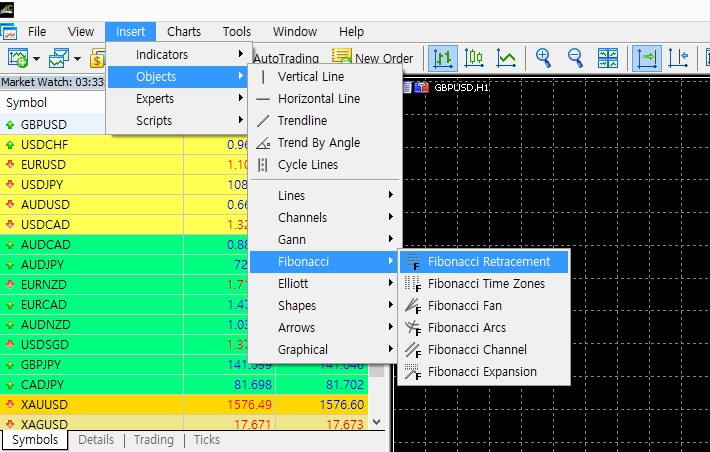2. How to apply them on chart ?

You will apply Fibonacci Retracement Chart Object using mouse with Click and Hold move. It is not double click but you will click at first point and then you will hold until the second point.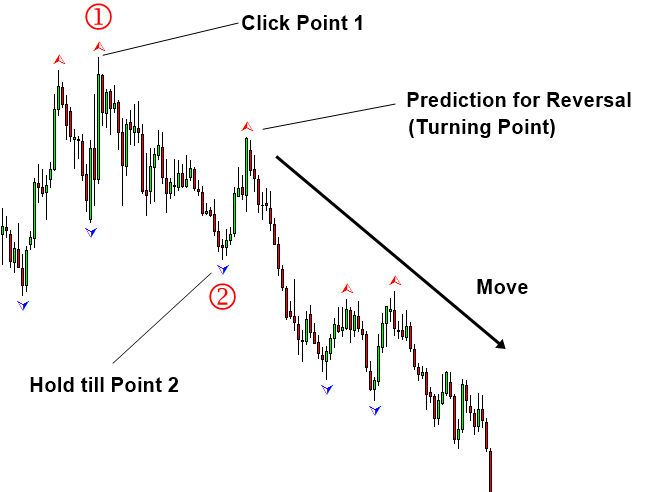3. Theoretical and Practical Points to note

Theoretically, one Fibonacci Retracement is equivalent to one Fractal Triangle made up from 3 points. You need to have a habit of coordinating fractal triangles into points for more advanced training later. In RECF notation, Fibonacci Retracement of 61.8% can be expressed as :

R: 0.618 or R: 61.8%

Never apply Fibonacci Retracement alone in your trading. Always use it together with Secondary Confirmation after checking surrounding market condition.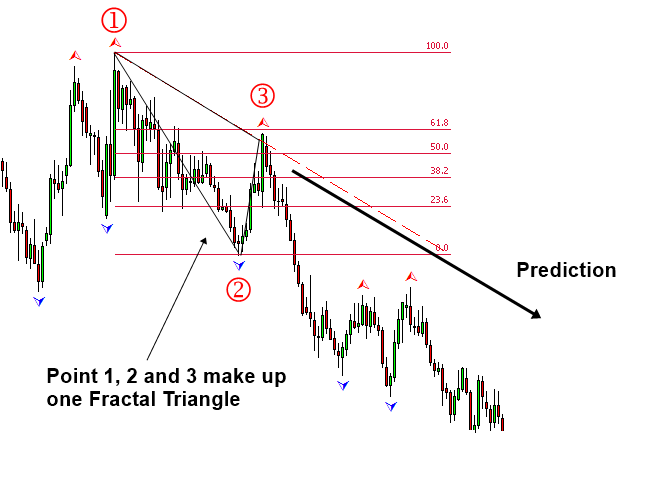4. Configure Fibonacci Ratios in Fibonacci Retracement

Typically, platform provider will provide the default ratios for Fibonacci Retracement. However, you can change the default ratios to anything you want. To do so, double click on Fibonacci Retracement in Chart. Click on Properties of Fibonacci Retracement.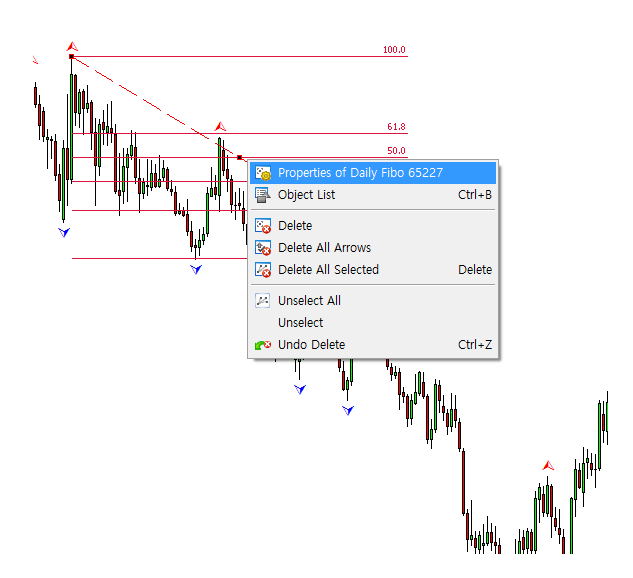With Property Window pop up, you can edit the Fibonacci Ratio + line properties of each Fibonacci Retracement levels.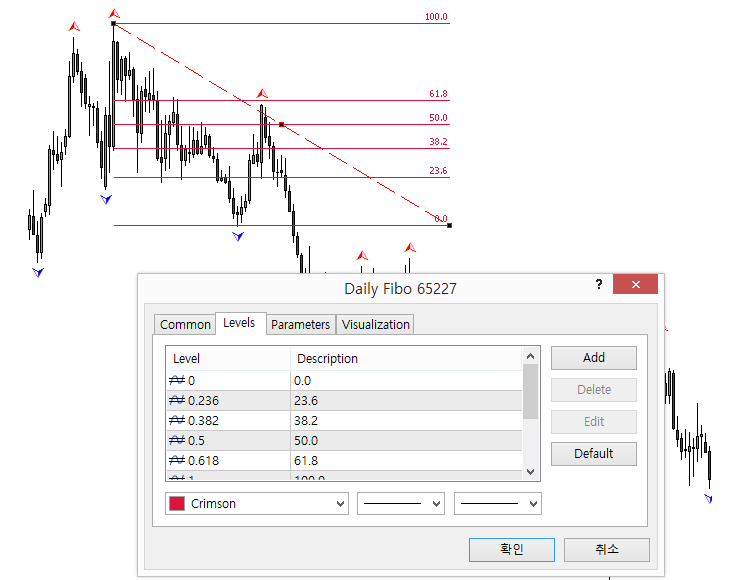Below is the landing page for Harmonic Pattern Plus, Harmonic Pattern Scenario Planner and X3 Chart Pattern Scanner in MetaTrader. All these products are also available from www.mql5.com too.